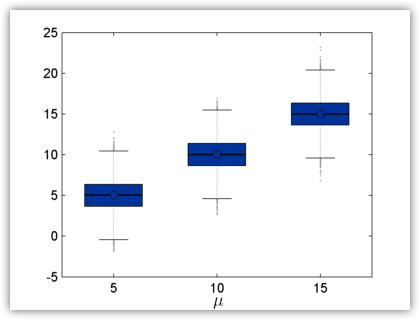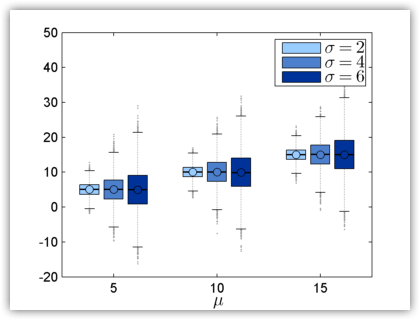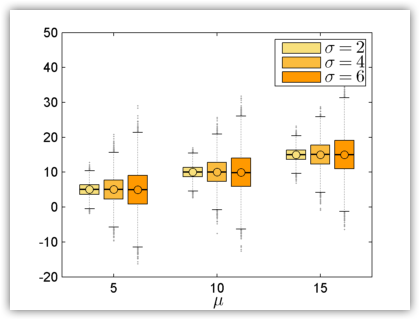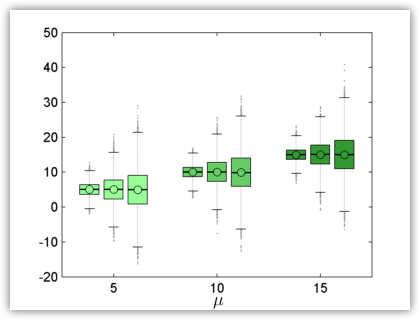# Advanced Box Plot for Matlab

## Overview

To illustrate the statistical properties of large data sets, Matlab includes a builtin box plot function. Although this function is a suitable tool in everyday use, some circumstances may require a more powerful alternative. These include the addition of the sample mean to the boxplot data, the grouping of data based on a given criterion and customzied coloring for publishing. To address these requirements, in this page, we introduce an advanced box blot function designed for Matlab power users.

Beta 1 version as of September 27, 2012.

## Usage

To plot an advanced boxplot, call the following function:

aboxplot(x);

or:

aboxplot(x, 'argument_name', argument_value, ...);

where x may be:

• A two-dimensional n x m numeric matrix. The figure will contain a number of m box plot bars, equal to the number of columns. Each box plot bar will use the n values from the corresponding data set.
• A three-dimensional p x n x m numeric array. The figure will contain a m groups of p box plot bars each. Each box plot bar will use the n values from the corresponding data set.
• An array of p cells, where each cell contains a n x m numeric matrix. The figure will contain a m groups of p box plot bars each. Each box plot bar will use the n values from the corresponding data set.

Currently, the following optional arguments are supported:

Argument name Argument description
'Colorgrad'
A text string representing the color gradient applied to the box plot bars. Currently, the following colors are supported:

'blue_down'
'blue_up'
'green_down'
'green_up'
'orange_down'
'orange_up'
'red_down'
'red_up'

'Colorrev'
A boolean value:
• if false, the color gradient applies across the m box plot bars within a group (default);
• if true, the color gradient applies across the p groups.
'Colormap'
A color matrix, which specifies custom colors for the box plot bars.
'Labels'
The X-axis labels, corresponding to the m groups.
'OutlierMarker'
The marker used to plot the outliers.
'OutlierMarkerSize'
The size of the marker used to plot the outliers.
'OutlierMarkerEdgeColor'
The edge color of the marker used to plot the outliers.
'OutlierMarkerFaceColor'
The face color of the marker used to plot the outliers.
'WidthE'
A numeric value in the interval (0, 1), represeting the width of the whisker edge, relative to width available to an individual box plot bar.
'WidthL'
A numeric value in the interval (0, 1), represeting the width of the box plot group, relative to width available to an individual box plot group.
'WidthS'
A numeric value in the interval (0, 1), represeting the width of the box plot bar, relative to width available to an individual box plot bar.

## Tutorial

To demonstrate the power of the advanced box plot function, we include a walkthrough that covers the most common features.

### Single Box Plot

This example plots three data sets of normally distributed random variables, x1, x2 and x3 with a mean of 5, 10 and 15, respectively. The data sets must have the same an equal number of elements (10000) and are concatenated in a 10000 x 3 matrix.

x1 = normrnd(5,2,10000,1); % First data set of 10000 values with mean 5
x2 = normrnd(10,2,10000,1); % Second data set of 10000 values with mean 10
x3 = normrnd(15,2,10000,1); % Third data set of 10000 values with mean 15

x = cat(2,x1,x2,x3); % Concatenate the data sets in a 10000 x 3 matrix

xlabel('$\mu$'); % Set the X-axis labelSingle box plot

### Grouped Box Plot with 3D Array

This example plots three groups of tree data sets each, of normally distributed random variables. The data sets in each group have a mean of 5, 10 and 15, respectively. The standard deviation for each group is 2, 4 and 6, respectively.

There are two possibilities to plot grouped data sets. In the first, the data sets must have the same the number of elements (10000) and are concatenated in a 3 x 10000 x 3 array, where the first dimesion represents the group.

% First group of 3 data set with standard deviation 2
x1 = normrnd(5,2,10000,1);
x2 = normrnd(10,2,10000,1);
x3 = normrnd(15,2,10000,1);
% Second group of 3 data set with standard deviation 4
y1 = normrnd(5,4,10000,1);
y2 = normrnd(10,4,10000,1);
y3 = normrnd(15,4,10000,1);
% Third group of 3 data set with standard deviation 6
z1 = normrnd(5,6,10000,1);
z2 = normrnd(10,6,10000,1);
z3 = normrnd(15,6,10000,1);

% Concatenate the data sets from each group in a 10000 x 3 matrix
x = cat(2,x1,x2,x3);
y = cat(2,y1,y2,y3);
z = cat(2,z1,z2,z3);

% Concatenate the each group in a  3 x 10000 x 3 matrix
h = cat(1, reshape(x,[1 size(x)]), reshape(y,[1 size(y)]), reshape(z,[1 size(z)]));

xlabel('$\mu$'); % Set the X-axis label

legend('$\sigma=2$','$\sigma=4$','$\sigma=6$'); % Add a legendGrouped box plot with 3D array

### Grouped Box Plot with Cell Array

The second possibility is to use a cell array instead of a three dimensional array. The cell array has the advantage that it does not require the same number of elements for data sets in different groups. However, data sets in the same group must still have an equal number of elements.

% First group of 3 data set with standard deviation 2
x1 = normrnd(5,2,10000,1);
x2 = normrnd(10,2,10000,1);
x3 = normrnd(15,2,10000,1);
% Second group of 3 data set with standard deviation 4
y1 = normrnd(5,4,10000,1);
y2 = normrnd(10,4,10000,1);
y3 = normrnd(15,4,10000,1);
% Third group of 3 data set with standard deviation 6
z1 = normrnd(5,6,10000,1);
z2 = normrnd(10,6,10000,1);
z3 = normrnd(15,6,10000,1);

% Concatenate the data sets from each group in a 10000 x 3 matrix
x = cat(2,x1,x2,x3);
y = cat(2,y1,y2,y3);
z = cat(2,z1,z2,z3);

h = {x;y;z}; % Create a cell array with the data for each group

xlabel('$\mu$'); % Set the X-axis label

legend('$\sigma=2$','$\sigma=4$','$\sigma=6$'); % Add a legendGrouped box plot with cell array

Blue is the default color for the advanced box plot, and the function uses a color gradient for the data sets in individual groups. The colorgrad option enables the user to change the default color gradient with seven other possible alternatives.

...
...### Coloring Groups

By default, the advanced box plot applies the color gardient across the data sets in individual groups. Use the colorrev option set to true to apply the gradient across groups.

...